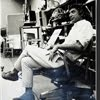## Eugene Ray SDSU 1970'sEugene Ray 1970's. photo credit: Tom Davis

## Saturday, March 30, 2013

### BIOMORPHIC VOLUMES OFFER FLUID RADIANT ENERGY SPACE >ancient alien radiant volumes >atlantis/sumeria/egypt/malta ^^^^^^^^^^^^^^^^^^^^ BIOTRONIC ENERGY VORTEXES > as found in ancient architecture ^^^^^^^^^^^^^^^^^^^^^ CONICAL BIOTRONIC RADIANCE as found in ancient architecture ^^^^^^^^^^^^^^^^^^^^^ THE GODS (ALIENS) MACHINES the research of wun chok bong^^^^^^^^^^^^^^
>my prototype designs using<
+CONES & SPHERES+
>are so ancient they are in the<
+"AVANT-GARDE"+
^^^^^^^^
>visionary french term<
>relished by degruy<
>ancestors who<
^^^^^^^^
+BUILT A DOME SUGAR MILL+
>new orleans<
^^^^^^^^^^^^^
(>giving them an award winning<
>quality sugar crop production<)
^^^^^^^^^^^^^^^

+GRATITUDE+
>this report on the research of<
+WUN CHOK BONG+
>is dedicated to sdsu alums<
>kevin and jasmin brekke<
^^^^^^^^^^^^^

+BIOTRONIC VOLUMES+
>as i have written before the<
+BRILLIENT RESEARCH+
>of wun chok bong corroborates<
>much of my own research<
>in biotronic energy<
^^^^^^^^^^

+THE SUITCASE OF BOOKS<
>carried to my uk lectures<
>would have been<
+UNECESSARY+
^^^^^^^

+"THE GODS MACHINES"+
>wun chok bongs invaluable book<
>would have been required<
+S T U D Y+
>in my classes at sdsu<
^^^^^^^^^^^

+TONIGHT'S PHOTOS+
>biomorphic architectural volume<
>generated by conical devices<
^^^^^^^^^^^^^^^
+GRAPHICS+
>all illustrations from the book<
+"THE GODS'S MACHINES"+
>by wun chok bong<
^^^^^^^^^

#1)        +CONICAL DEVICES+
>turned ancient neolithic temple<
+S P H E R I C A L+
+VORTEXES+
^^^^^
#2)               +TARXIEN+
>temple conical power generator<
+MALTA 2500 BC+
>with energy vortex reliefs<
+IDEOGRAMS+
>on the lateral walls<
^^^^^^^^^^
#3)           +TWO VIEWS+
>wall view on the left depicts the<
>of spiraling energy as seen in<
>the plan view circular<
+VOLUMES+
>in the temple (right)<
^^^^^^^^^^
+CONICAL DEVICE+
>activating the force fields<
>located in the center<
^^^^^^^^^^^

#4)         +VORTEX RELIEF+
>with basin that held the central<
+CONICAL DEVICE+
>as seen by david childress in<
>his research on malta<
^^^^^^^^^^^

#5)         +MALTA TEMPLES+
>three different malta temple plans<
>with conical energy generators<
+VORTEXES+
>in the domical volumes<
^^^^^^^^^^^^

+"ANCIENT ALIENS"+
>the great history channel television<
>series was on tonight w/ much<
>data to support the many<
+AVANT-GARDE+
>concepts i present here<
^^^^^^^^^^^^^

+INCREASINGLY+
>technical experts like chris dunn<
>the master machinist attest<
>to obvious conclusions<
^^^^^^^^^^^^
+EXTRATERRESTRIAL+
+INTERVENTION+
^^^^^^^^
>was the source of much design<
>and early earth construction<
+A M E N+
^^^^^

>gene ray, lajolla, california<

>eugene ray, mfa, architect<
>professor emeritus, sdsu<
^^^^^^^^^^^^^